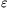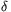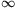Mathematical and Physical Journal
for High Schools
Issued by the MATFUND Foundation
 Already signed up? New to KöMaL?

#Problem A. 552. (January 2012)

A. 552. Prove that for an arbitrary sequenceof nonnegative real numbers and>0 there exist infinitely many positive integers n for which.

(Schweitzer-competition, 2011)

(5 pont)

Deadline expired on February 10, 2012.

Solution. We prove by contradiction. Suppose that there is a sequenceand>0 for which(1)

holds with finitely many exception. As a simple corollary of (1) we have

 4an(1-an-1)>1; (2)

it follows that an>0 and an-1<1. Moreover, from the AM-GM inequality we getso an>an-1; the sequence increases from the index n0.

By the monotonicity and boundedness the sequence converges. We show that its limit is. Let; from (2) we see that(2A-1)20,

therefore. Hence,.

Define. This sequence decreases from the index n0, and it converges to 0. Transforming (1), for n>n0 we have(3)

Summing up (3), for n>n0 we get a lower bound for xn:(4)

In the rest of the solution we consider the properties of the sequence (n+1)xn. We distinguish two cases.

Case 1: the sequence (n+1)xn has a finite accumulation point. Sinceexcept for finitely many indices, all accumulation points lie in the interval. It is well-known that there is a minimal accumulation point; this point is called as the limes inferior of the sequence. Denote by c the smallest accumulation point. Then there is a sequenceof indices such that.

Since there is no smallest accumulation point than c, for all>0, by the Bolzano-Weierstrass theorem, (n+1)xn>c-holds except for finitely many indices. Substuting this into (3) and summing up again, we obtain that for nk>n0 andc,From the transition kwe getThen from+0, we obtainCase 2: The sequence (n+1)xn has no accumulation point. By the Bolzano-Weierstrass theorem and the positivity this means that for all real K, (n+1)xnwith finitely many exceptions, so (n+1)xn. Then there is an index n>n0 such that 1<nxn-1<(n+1)xn. Applyinig (3),we get a contradiction, since both nxn-1-(n+1)xn and 1-2(n+1)xn are negative.

Remark. The termin the problem statement is important. Ommiting this quantity the statement would be false. For example, for the sequence,### Statistics:

 6 students sent a solution. 5 points: Ágoston Tamás, Mester Márton, Omer Cerrahoglu, Strenner Péter. 1 point: 2 students.

Problems in Mathematics of KöMaL, January 2012